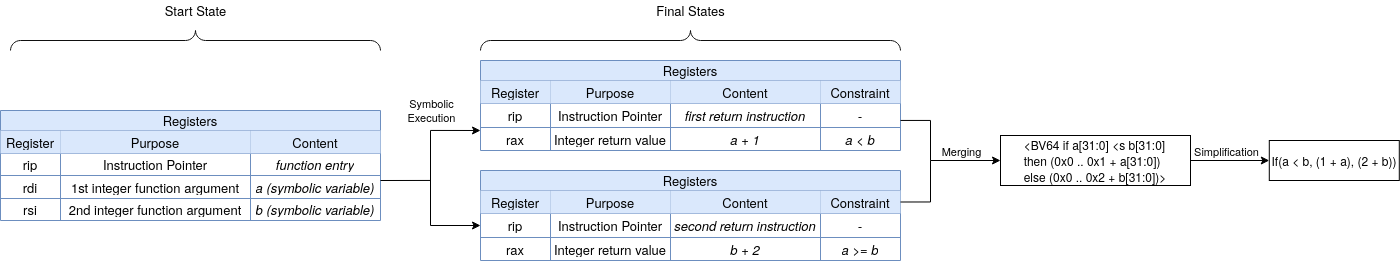# Introduction

This post provides an overview of a paper, PERFUME, we submitted to the CheckMATE 2021 workshop. In PERFUME, we discuss an approach to extract mathematical expressions from binary executables in order to present these to a subject matter expert. This allows such a subject matter expert to reason about these expressions without requiring expertise in reverse engineering.

The approach introduced in PERFUME relies on symbolic execution and machine translation. Symbolic execution is used to extract symbolic expressions of the computations performed by the binary instructions. Symbolic execution has the advantage of producing expressions relatively free from the other noise occurring in binary executables, such as register and memory manipulation. Once such a symbolic expression is extracted, machine translation is used to refine this expression to make it more understandable by humans. The full paper can be read here.

# Symbolic Execution

We perform symbolic execution on a target function, chosen by the analyst. At the start of symbolic execution, we setup a program state with symbolic variables, named by the analyst, to use as input parameters for the target function. After symbolic execution completes, we extract the expression of the return value of the function. We assume this expression captures the computation performed in the function.

We show an example on the following function.

int f(int a, int b) {
if (a < b) {
return a + 1;
} else {
return b + 2;
}
}


The figure below shows the process of performing symbolic execution on this function.## Function Summarization

We use a technique to simplify symbolic expressions called function summarization. This technique summarizes the subexpressions of a callee function (of the target function) by replacing these with a symbolic function. The symbolic expressions that are passed to the callee function during symbolic execution are included as the parameters of the symbolic function. This helps to create a more succinct and understandable symbolic expression.

We use the following code as an example.

float f(float a, float b) {
return a * sx_floor(b);
}


The function sx_floor calculates the mathematical floor of its input parameter. If we extract the symbolic expression while summarizing this function, we extract the expression a * floor(b). However, if we do not summarize this function, we extract the following expression.This clearly shows the benefit that function summarization provides in terms of understandability.

# Machine Translation

After extracting symbolic expression, we use machine translation to simplify these further. To this end we use BLEU, the standard evaluation metric for machine translation. We notice, however, that while BLEU works well for natural language, it does not capture the equivalence between mathematical statements well. We are currently investigating other evaluation metrics.